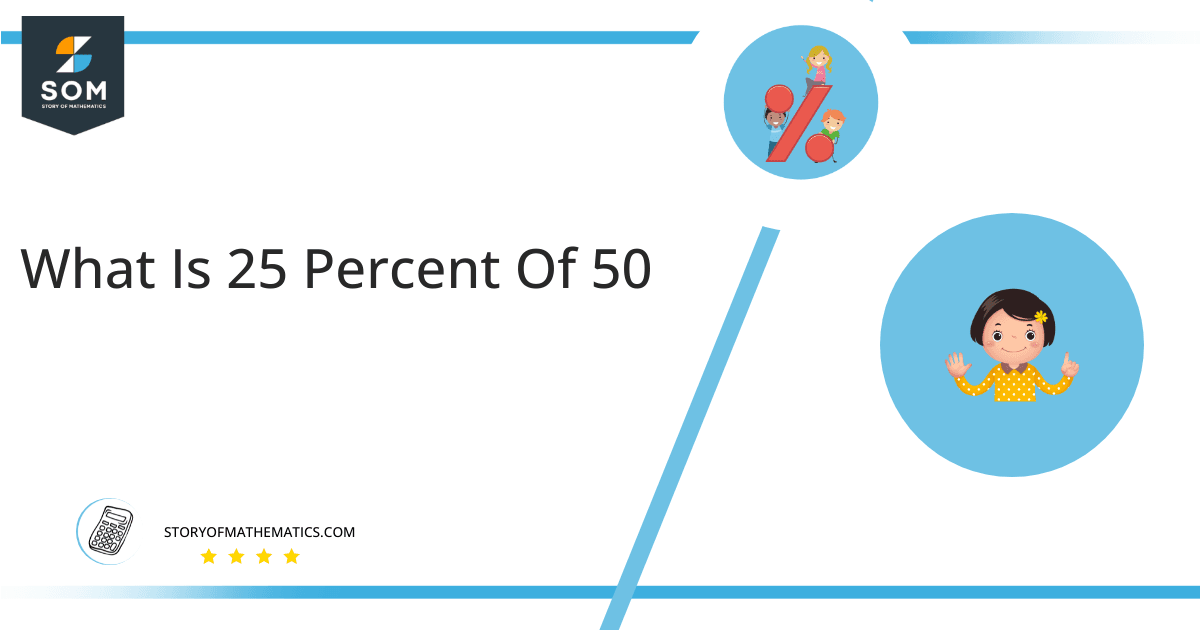# What Is 25 Percent of 50 + Solution With Free Steps25 percent of 50 is 12.5. To get this answer, we need to multiply 0.25 by 50.

Various practical examples follow the problems given in this article. Let’s suppose you want to buy your favorite dress from your favorite brand and suddenly you come to know that the brand announce end season sale of 25 percent off on all their items. You go shopping and your favorite dress costs 50 dollars. Because of the sale, you get 25% off and you want to estimate the amount you save. You estimate the 25 percent of 50 by multiplying 0.25 by 50. The output is 12.5 which means that you save 12.5 dollars. Many other real-life problems are related to this article.

This article presents a more organized solution to this problem with step by step solution.

## What Is 25 Percent of 50?

25 percent of 50 equals 12.5. To calculate the answer, we need to multiply 0.25 by 50. There are a lot of ways to solve percentage problems like this.

## How To Calculate 25% of 50?

Following are the steps used to solve the 25 percent of 50.### Step 1

In the given scenario, the output value is 50. The unknown value is considered as x.

### Step 2

The output value is also written as

50 = 100%

In the same way

x = 25%

### Step 3

Now we have two equations

Equation 1 is given as

50 = 100%

Equation 2 is given as

x = 25%

### Step 4

On dividing the pair of a simple equation, we get

50% / x% = 100/ 25

### Step 5

By taking reciprocal of both sides of the equations. We get

x% /50% = 25 / 100

### Step 6

Simplify, the above simple equation given the answer

x = 12.5

Therefore, 25 percent of 50 is 12.5. The pie chart for the following problem is given as :Figure 1: 25 percent of 50 on Pie-chart

The pie chart represents 25 percent of 50. The 25% of 50 is 12.5 which is shown in the blue area. The total area is 50. The remaining is 75% which is 37.5 as shown in the red area of the graph.

The definition of a percentage is the normalized value of a given number relative to its maximum value, where all potential values ​​of a given number can be mapped onto a scale of 0 to 100.

All the Mathematical drawings/images are created using GeoGebra.